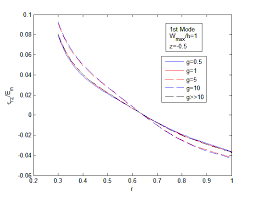## How to Calculate and Solve for Well, External and Dimensionless Radius | Nickzom CalculatorThe image above represents dimensionless radius.

To compute for the dimensionless radius, two essential parameters are needed and these parameters are external radius (re) and well radius (rw).

The formula for calculating the dimensionless radius:

rD = re / rw

Where;

rD = Dimensionless Radius
re = External Radius
rw = Well Radius

Let’s solve an example;
Given that the external radius is 18 and the well radius is 16.
Find the dimensionless radius?

This implies that;

re = External Radius = 18
rw = Well Radius = 16

rD = re / rw
rD = 18 / 16
rD = 1.125

Therefore, the dimensionless radius is 1.125.

Calculating for the External Radius when the Dimensionless Radius and the Well Radius is Given.

re = rD x rw

Where;

re = External Radius
rD = Dimensionless Radius
rw = Well Radius

Let’s solve an example;
Given that the dimensionless radius is 9 and the well radius is 20.
Find the external radius?

This implies that;

rD = Dimensionless Radius = 9
rw = Well Radius = 20

re = rD x rw
re = 9 x 20
re = 180

Therefore, the external radius is 180.